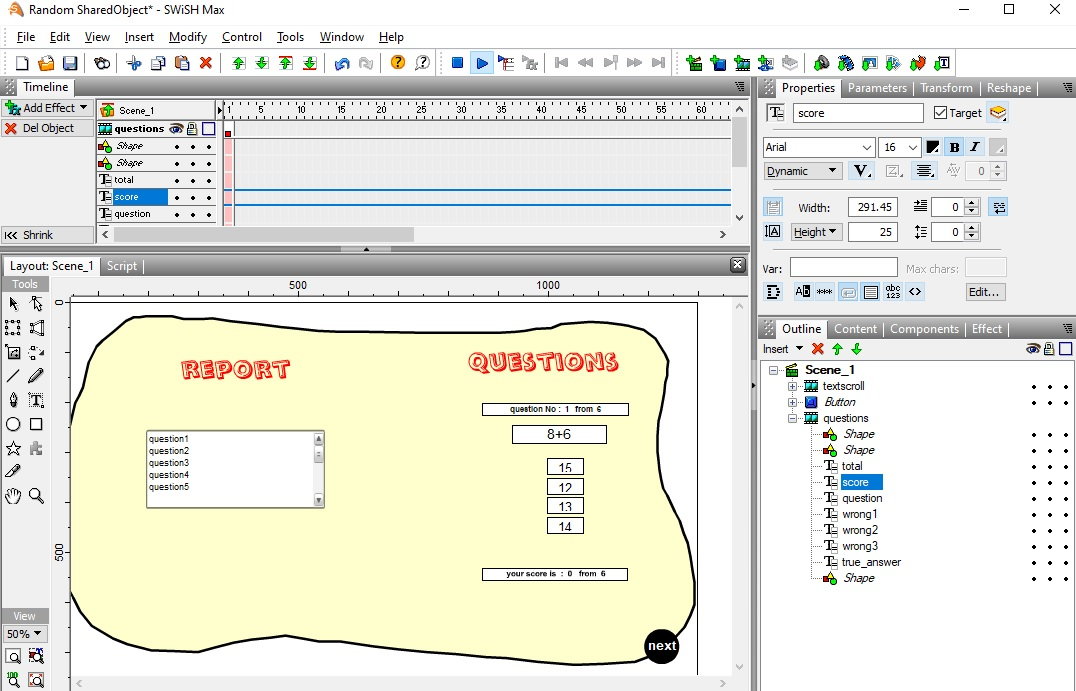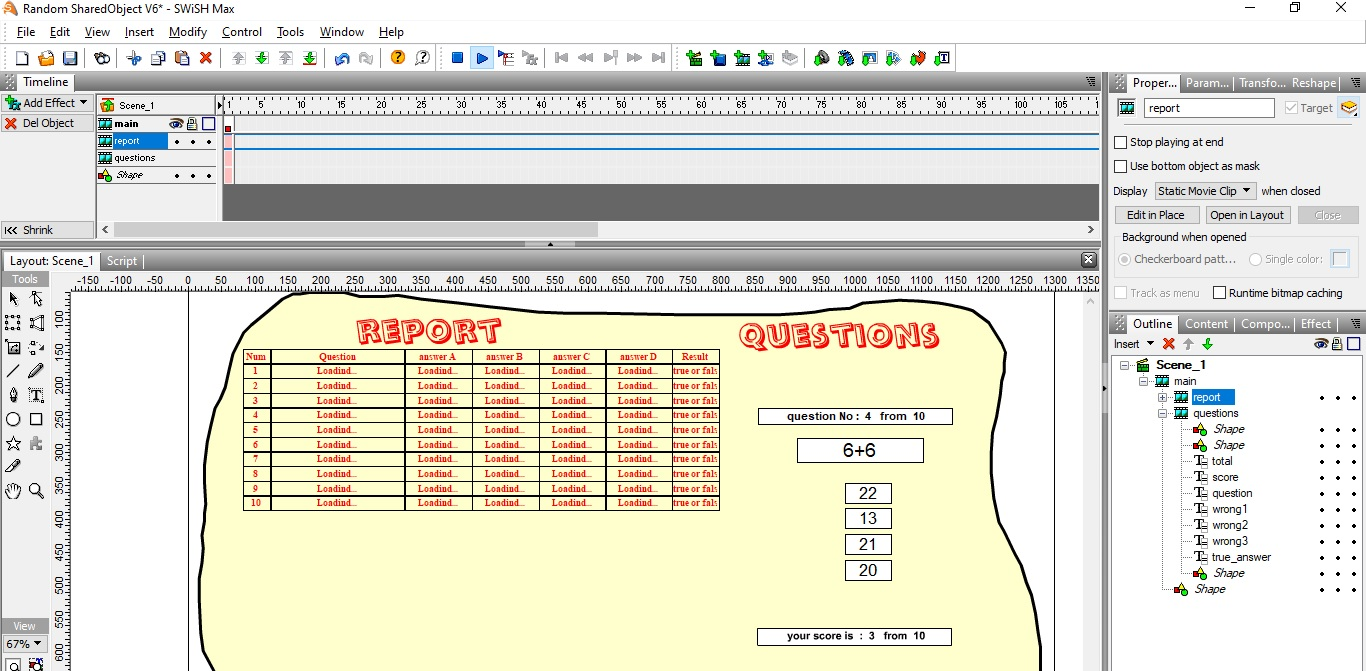A Flash Developer Resource Site

1. ## Random SharedObject

hello all

this is my new project in swishmaxThe file loads randomly questions from a text file
The student answers only 5 questions per day
How to save the questions answered in the report ( textscroll ) by SharedObject method or any

and On the next day, when you open the file again, it completes the questions without repeating the questions that were answered

and add a new data in the report

and so on

the report contains the question and 4 answered and true or false

swishmax file : https://app.box.com/s/0g84psgzvj5ww1cfzn4bhdan1fgbmvw1

thanks for helpReply With Quote

2. https://open-flash.github.io/mirrors...redObject.html There are some code examples you can get a start with.Reply With Quote

3. thanks swake
I read this but I don't know how to do it
i need your experience to make this project together as you helped me in the pastReply With Quote

4. Hello, I added an open day which means if the settings.txt says 11/2/2022 & computer date says 11/1/2022, tomorrow it will be unlocked.

To show a snippet, I just send the openDay function the open date from settings.txt
PHP Code:
``` function openDay(a){ var temp_month=(a.split(";")) var temp_day=(a.split(";")) var temp_year=(a.split(";")); //temp_month + " " +temp_day + " " + temp_year + " " + //_root.questions.data_field3.text=temp_month==(current_month_number) //+ " " +temp_month + " " +temp_day + " " + temp_year + " " + current_month_number+" " + current_day_number + " " + current_year_number if(temp_year==(current_year_number)&&temp_day==(current_day_number)&&temp_month==(current_month_number)){ return("unlocked"); } if(temp_year<(current_year_number)){ return("unlocked"); } if(temp_month<(current_month_number)&&temp_year<=(current_year_number)){ return("unlocked"); } if(temp_day<=(current_day_number)&&temp_month<=(current_month_number)&&temp_year<=(current_year_number)){ return("unlocked"); } return("locked"); }  ```Reply With Quote

5. welcome AS3.0

let's start together again

i have an idea

We create an array when the file is run the first time and store the questions numbers in it
Then the questions are called in the following through this array
I made this code, but I need your expertise to complete the code

PHP Code:
``` onSelfEvent (load) {      var open:SharedObject = SharedObject.getLocal("storedFile");    open.data.array || []; //  array to save questions numers  if (open.data.first_run ==undefined){;   trace("first time")    open.data.first_run =0 // to delete load questions again in the next run  // start to load questions and answers from txt file      loadV = new LoadVars();                             MyArray = new Array();     loadV.load("settings.txt");       loadV.onData = function(s) {         p = s.split('&');                       for(i=1; i < p.length; i++) {                         MyArray[i]=p[i].split('@');                                                                                                                }        var numbers: Array = new Array();         for (var i: int = 1; i < p.length; i++) {          numbers.push(i);                 }        numbers = numbers.sort(function (a:int, b:int):int { return Math.random() > .5 ? -1 : 1; });     trace(numbers)     }      //end load questions and answers from txt file     // here how to save these random numbers in (open.data.array SharedObject  )    // to use it in scand open   }   else if (open.data.first_run ==0){ // if this is the scand open     trace("scand open");     //here how to load and complete the questions              }          open.flush()      //open.clear();   // enable this code to delete all stored data to check your code again } onSelfEvent (enterFrame) {            /*  // i disable this code until any help about this idea         question.text = MyArray[numbers[num]].split("q"+numbers+"=");         wrong1.text = MyArray[numbers[num]].split("q"+numbers+"=") ;         wrong2.text = MyArray[numbers[num]].split("q"+numbers+"=") ;         wrong3.text = MyArray[numbers[num]].split("q"+numbers+"=") ;    true_answer.text = MyArray[numbers[num]].split("q"+numbers+"=") ;    total.text=" question No :  " add (_root.questions.num+1) add "   from  " add  (_root.questions.p.length-1)       score.text=" your score is  :  " add (int(_root.questions.scorePOINT)) add "   from  " add  (_root.questions.p.length-1)         */                          } onFrame (1) { stop(); }  ```

swishmax file :https://app.box.com/s/c6lc3fd2zsm9ysprswhw9lcrgu0cvq4bReply With Quote

6. hello alli have succeeded in :

1- Random numbers are stored
3- Complete the questions at the last question answered
4- Create a report table.

but

There are still many things that need help:

1- Is there a way to make the report in a professional and easy way?

2- How to change the position of the answers in a random way(This of course will change the position of the answers in the report)

questions script:

PHP Code:
``` onSelfEvent (load) {           var open:SharedObject = SharedObject.getLocal("storedFile");    open.data.Questions || []; // to save questions and answers    open.data.array || []; // to save random number       if (open.data.first_run ==undefined){;   trace("first time");    open.data.first_run =0 // to delete load questions again in the next run    open.data.QuestionNum= 0 // to save questions answered      open.data.POINT=0;    //trace(open.data.QuestionNum)  // start to load questions and answers from txt file      loadV = new LoadVars();                             MyArray = new Array();     loadV.load("settings.txt");       loadV.onData = function(s) {         p = s.split('&');                       for(i=1; i < p.length; i++) {                         MyArray[i]=p[i].split('@');                                                                                                                }         open.data.Questions=MyArray;        var numbers: Array = new Array();         for (var i: int = 1; i < p.length; i++) {          numbers.push(i);                 }        numbers = numbers.sort(function (a:int, b:int):int { return Math.random() > .5 ? -1 : 1; });     //trace(numbers);     open.data.array=numbers;     trace(open.data.array);      trace(open.data.QuestionNum);     }     }   else if (open.data.first_run ==0){ // if this is the scand open     trace("scand open");     //here how to load and complete the questions       trace(open.data.array);     trace(open.data.QuestionNum);      }          open.flush()      //open.clear();   // enable this code to delete all stored data to check your code again TrueAnswe () } onSelfEvent (enterFrame) {     if(open.data.first_run ==0){TrueAnswe ()}                         } onFrame (1) { stop(); } function TrueAnswe () {                  question.text = open.data.Questions[open.data.array[open.data.QuestionNum]];         wrong1.text = open.data.Questions[open.data.array[open.data.QuestionNum]];         wrong2.text = open.data.Questions[open.data.array[open.data.QuestionNum]];         wrong3.text = open.data.Questions[open.data.array[open.data.QuestionNum]] ;         true_answer.text = open.data.Questions[open.data.array[open.data.QuestionNum]] ;         total.text=" question No :  " add (open.data.QuestionNum+1) add "   from  " add  (open.data.array.length)            score.text=" your score is  :  " add (open.data.POINT) add "   from  " add  (open.data.array.length) }  ```

report script:

PHP Code:
``` onFrame (1) {           stop();     for( var i:int = 0; i < _root.main.questions.open.data.array.length+1 ; ++i ){  // create question number table          this.createTextField("num"+i, this.getNextHighestDepth(), 50, , 40,22);             this["num"+i]._y=(i*22)+1     this["num"+i].border = true;     this["num"+i].text = String(i);     this["num"+0].text = "Num";     this["num"+i].selectable=false // create question table          this.createTextField("Question"+i, this.getNextHighestDepth(), 92, , 200,22);             this["Question"+i]._y=(i*22)+1     this["Question"+i].border = true;     this["Question"+i].text = "Loadind...";     this["Question"+0].text = "Question";     this["Question"+i].selectable=false;     // create Answer A table          this.createTextField("answerA"+i, this.getNextHighestDepth(), 293, , 100,22);             this["answerA"+i]._y=(i*22)+1     this["answerA"+i].border = true;     this["answerA"+i].text = "Loadind...";     this["answerA"+0].text = "answer A";     this["answerA"+i].selectable=false // create Answer A table          this.createTextField("answerB"+i, this.getNextHighestDepth(), 393, , 100,22);             this["answerB"+i]._y=(i*22)+1     this["answerB"+i].border = true;     this["answerB"+i].text = "Loadind...";     this["answerB"+0].text = "answer B";     this["answerB"+i].selectable=false     // create Answer C table          this.createTextField("answerC"+i, this.getNextHighestDepth(), 493, , 100,22);             this["answerC"+i]._y=(i*22)+1     this["answerC"+i].border = true;     this["answerC"+i].text = "Loadind...";     this["answerC"+0].text = "answer C";     this["answerC"+i].selectable=false // create Answer D table          this.createTextField("answerD"+i, this.getNextHighestDepth(), 593, , 100,22);             this["answerD"+i]._y=(i*22)+1     this["answerD"+i].border = true;     this["answerD"+i].text = "Loadind...";     this["answerD"+0].text = "answer D";     this["answerD"+i].selectable=false // create Result table  this.createTextField("Result"+i, this.getNextHighestDepth(), 693, , 70,22);             this["Result"+i]._y=(i*22)+1     this["Result"+i].border = true;     this["Result"+i].text = "true or false";     this["Result"+0].text = "Result";     this["Result"+i].selectable=false     var myformat:TextFormat = new TextFormat(); myformat.size=13 ; myformat.color = 0xFF0000; myformat.bold=true; myformat.align="center";   this["Question"+i].setTextFormat(myformat); this["Question"+0].setTextFormat(myformat); this["num"+i].setTextFormat(myformat); this["num"+0].setTextFormat(myformat); this["answerA"+i].setTextFormat(myformat); this["answerA"+0].setTextFormat(myformat); this["answerB"+i].setTextFormat(myformat); this["answerB"+0].setTextFormat(myformat); this["answerC"+i].setTextFormat(myformat); this["answerC"+0].setTextFormat(myformat); this["answerD"+i].setTextFormat(myformat); this["answerD"+0].setTextFormat(myformat); this["Result"+i].setTextFormat(myformat); this["Result"+0].setTextFormat(myformat);     } }  ```

Random SharedObject V4 file : https://app.box.com/s/xu0uolljdnp4mkd440of1bb8dmnu2ufdReply With Quote

7. Hello for #2 I added a function called randomizeOrder() that will reorder the x & y coordinates by memorizing the original way they were placed in another array called coordinates.

Inside of the true_answer button you can call this each time, to create a new order.
PHP Code:
``` _root.main.questions.randomizeOrder();  ```
The randomBetween function is inside of the questions section.
PHP Code:
``` function randomBetween(a,b){ return(Math.floor(Math.random()*(b-a+1))+a); }  ```
The randomizeOrder function is inside of the questions section.
PHP Code:
``` function randomizeOrder(){     var main_array:Array=new Array(wrong1,wrong2,wrong3,true_answer)     var main_array2:Array=new Array(wrong1,wrong2,wrong3,true_answer)     var coordinates:Array=new Array();     var numbers:Array=new Array(0,1,2,3);     var new_order:Array=new Array();          for(var i=0;i<4;i++){     coordinates.push(main_array[i]._x);         coordinates.push(main_array[i]._y);      new_order.push(numbers.splice(randomBetween(0,numbers.length-1),1));     }     var pos=0 for(var i=0;i<4;i++){ main_array[new_order[i]]._x=coordinates[pos] main_array[new_order[i]]._y=coordinates[pos+1] pos+=2 } }  ```Reply With Quote

8. thanks AS3.0

my solution is :

PHP Code:
``` function postion () {      var i:int=int(Math.randomRange(1,5)) ;     if(i==1){                 wrong1._y = 300.05;          wrong2._y =338.95 ;          wrong3._y =377.9 ;     true_answer._y = 416.8 ;                }         else if(i==2){                 true_answer._y = 300.05;          wrong3._y =338.95 ;          wrong2._y =377.9 ;          wrong1._y = 416.8 ;                }         else if(i==3){                 wrong1._y = 300.05;          true_answer._y =338.95 ;          wrong2._y =377.9 ;          wrong3._y = 416.8 ;                }         else if(i==4){                 wrong3._y = 300.05;          wrong1._y =338.95 ;     true_answer._y =377.9 ;          wrong2._y = 416.8 ;             }         }  ```

now can you help me to make the reportReply With Quote

9. Hello, I have created a solution to fill the report as the questions get answered.

So I took some of the program from the report & used it for the TrueAnswe function at the questions section.

In the TrueAnswe function if _root.attempt==1 & the text field wasn't already set as incorrect, we can put it as correct.
Each button press will increase the _root.attempt number, if it is only 1 attempt we can save the answer as a correct answer.
PHP Code:
```    if(_root.attempt==1&&  _root.main.questions.open.data.Questions[open.data.QuestionNum]!="incorrect"){   _root.main.questions.open.data.Questions[open.data.QuestionNum]="correct";    _root.main.report["Result"+(open.data.QuestionNum)].text ="correct";     _root.attempt=0  //Once you are on the next question it will reset _root.attempt to equal 0    }else if(_root.attempt>1&&_root.main.report["Result"+(open.data.QuestionNum)].text!="correct"&&_root.main.report["Result"+(open.data.QuestionNum)].text!="Result"){         _root.main.questions.open.data.Questions[open.data.QuestionNum]="incorrect";    _root.main.report["Result"+(open.data.QuestionNum)].text ="incorrect";    }  ```
Once we reach the final question:
1.)Should I enlarge the report for printing? btw printing can be done using the flash client. File > Print, or is this for a browser.
2.)Show a percentage?
3.)The report is only supposed to show up once the last question is reached?Reply With Quote

10. I had to fix the order for the report:

[open.data.QuestionNum-1] is 1-10 & increments as you answer each question to retrieve the scrambled array position in order for _root.main.questions.open.data.array, the scrambled array position at this level is for _root.main.questions.open.data.Questions which will give you the correct or incorrect type based on how the question was answered in ,  has "correct", "incorrect" or "null" saved.
PHP Code:
``` _root.main.questions.open.data.Questions[_root.main.questions.open.data.array[open.data.QuestionNum-1]]  ```Reply With Quote

11. Here is a bug fix from v7 that wasn't updating the data properly without the table being visible:Reply With Quote

12. No visible dataReply With Quote

13. Oh, that was unnecessary to place.

If you get to the final question you will see the report.Reply With Quote

14. very good

1-Is it possible for the question to appear in the report immediately after the answer?
2- Can the students answer be in a different color?
3- When the wrong choice is made, it moves to the next question
4- can you make a scroll for the reportReply With Quote

15. Hi, This answers question 1 & 3.

Inside of report we can go with having the borders set to false if the question is unanswered:
PHP Code:
``` this["num"+i].border = false; //hide border for unanswered questions  ```
I also sampled the background graphics hex value and set the text to be the same color as the background:
PHP Code:
``` myformat.color = 0xFFFFCC; //hide color for unanswered questions  ```
As open.data.QuestionNum number increases from selecting choices we can call trueAnswe at the questions section to enable .border to be true as well as set the text color to be red 0xFF0000:
PHP Code:
``` myformat.color = 0xFF0000; //set the color to red when a question gets answered _root.main.report["num"+i].border = true; //set the border to be visible when a question gets answered _root.main.report["Result"+i].border = true; _root.main.report["answerD"+i].border = true; _root.main.report["answerC"+i].border = true; _root.main.report["answerB"+i].border = true; _root.main.report["answerA"+i].border = true; _root.main.report["Question"+i].border = true;  ```
For buttons wrong1, wrong2, and wrong3 we can check if the current QuestionNum hasn't exceeded the array length _root.main.questions.open.data.array.length:
PHP Code:
``` if(_root.main.questions.open.data.QuestionNum< _root.main.questions.open.data.array.length){ _root.main.questions.open.data.QuestionNum +=1;  //increase the QuestionNum by one  _root.main.report["Result"+(open.data.QuestionNum)].text ="incorrect"; //set the text as incorrect _root.main.questions.open.data.Questions[_root.main.questions.open.data.array[open.data.QuestionNum-1]]="incorrect"; //save the data as incorrect _root.main.questions.TrueAnswe ()  //call TrueAnswe function to enable borders for the increased QuestionNum as well as set the color of the text to red: 0xFF0000 _root.main.questions.randomizeOrder(); //randomize the of the buttons for the next question _root.attempt=0 //reset the attempt number for the next question to start }  ```Reply With Quote

16.Reply With Quote

17. Hello, this version will save the arrangement.

I acquired the order by sorting A,B,C,D by _y coordinate which works just as good, If all the program was in 1 page it would be easier.Reply With Quote

18.Reply With Quote

19. Hi,

Above the TrueAnswe function at the questions section, I set 0x777788 to be the color of the selected answer.
PHP Code:
``` var selected_answer_color:Number=0x777788  ```
Inside of the TrueAnswe function at the questions section, this snippet will check all A,B,C, & D for each row on the report,  is where the selected answer is stored.
PHP Code:
``` if(_root.main.report["answerA"+i].text==_root.main.questions.open.data.Questions[_root.main.questions.open.data.array[i-1]]){ myformat.color =  _root.main.questions.selected_answer_color; _root.main.report["answerA"+i].setTextFormat(myformat);  } if(_root.main.report["answerB"+i].text==_root.main.questions.open.data.Questions[_root.main.questions.open.data.array[i-1]]){ myformat.color = _root.main.questions.selected_answer_color; _root.main.report["answerB"+i].setTextFormat(myformat);  } if(_root.main.report["answerC"+i].text==_root.main.questions.open.data.Questions[_root.main.questions.open.data.array[i-1]]){ myformat.color = _root.main.questions.selected_answer_color; _root.main.report["answerC"+i].setTextFormat(myformat);  } if(_root.main.report["answerD"+i].text==_root.main.questions.open.data.Questions[_root.main.questions.open.data.array[i-1]]){ myformat.color = _root.main.questions.selected_answer_color; _root.main.report["answerD"+i].setTextFormat(myformat);  }  ```
At the bottom of the script for each button, true_answer, wrong3, wrong2, & wrong1 this script will highlight the latest selected answer, Instead of using a full loop we can find the current selection with parseInt(_root.main.questions.open.data.QuestionNu m)-1.
PHP Code:
``` if(_root.main.report["answerA"+parseInt(parseInt(_root.main.questions.open.data.QuestionNum))].text==_root.main.questions.open.data.Questions[_root.main.questions.open.data.array[parseInt(_root.main.questions.open.data.QuestionNum)-1]]){ myformat.color = _root.main.questions.selected_answer_color; _root.main.report["answerA"+parseInt(parseInt(_root.main.questions.open.data.QuestionNum))].setTextFormat(myformat);  } if(_root.main.report["answerB"+parseInt(parseInt(_root.main.questions.open.data.QuestionNum))].text==_root.main.questions.open.data.Questions[_root.main.questions.open.data.array[parseInt(_root.main.questions.open.data.QuestionNum)-1]]){ myformat.color = _root.main.questions.selected_answer_color; _root.main.report["answerB"+parseInt(parseInt(_root.main.questions.open.data.QuestionNum))].setTextFormat(myformat);  } if(_root.main.report["answerC"+parseInt(parseInt(_root.main.questions.open.data.QuestionNum))].text==_root.main.questions.open.data.Questions[_root.main.questions.open.data.array[parseInt(_root.main.questions.open.data.QuestionNum)-1]]){ myformat.color = _root.main.questions.selected_answer_color; _root.main.report["answerC"+parseInt(parseInt(_root.main.questions.open.data.QuestionNum))].setTextFormat(myformat);  } if(_root.main.report["answerD"+parseInt(parseInt(_root.main.questions.open.data.QuestionNum))].text==_root.main.questions.open.data.Questions[_root.main.questions.open.data.array[parseInt(_root.main.questions.open.data.QuestionNum)-1]]){ myformat.color = _root.main.questions.selected_answer_color; _root.main.report["answerD"+parseInt(parseInt(_root.main.questions.open.data.QuestionNum))].setTextFormat(myformat);  }  ```Reply With Quote

20. Bug fix for wrong2:Reply With Quote

####Posting Permissions

• You may not post new threads
• You may not post replies
• You may not post attachments
• You may not edit your posts
•

 » Home » Movies » Tutorials » Submissions » Board » Links » Reviews » Feedback » Gallery » Fonts » The Lounge » Sound Loops » Sound FX » About FK » Sitemap